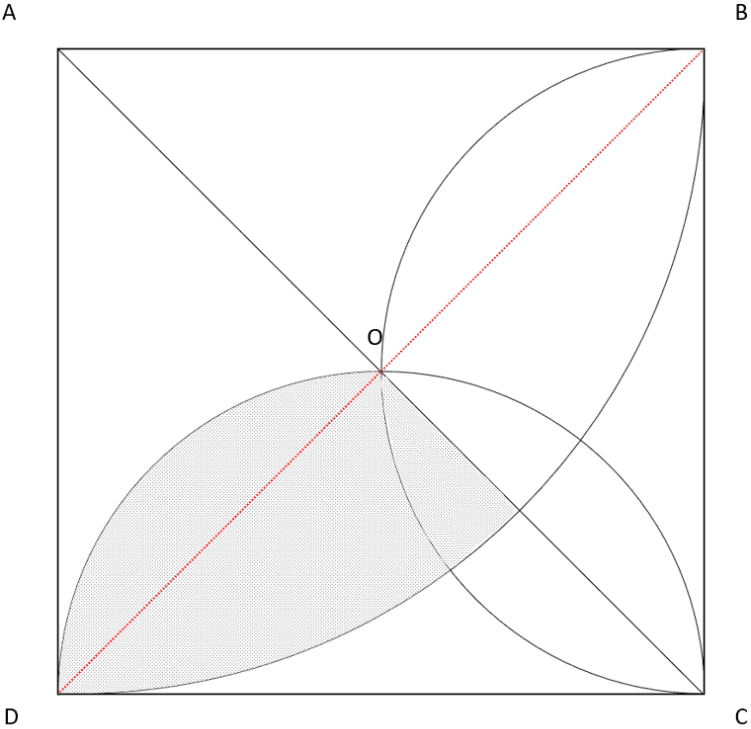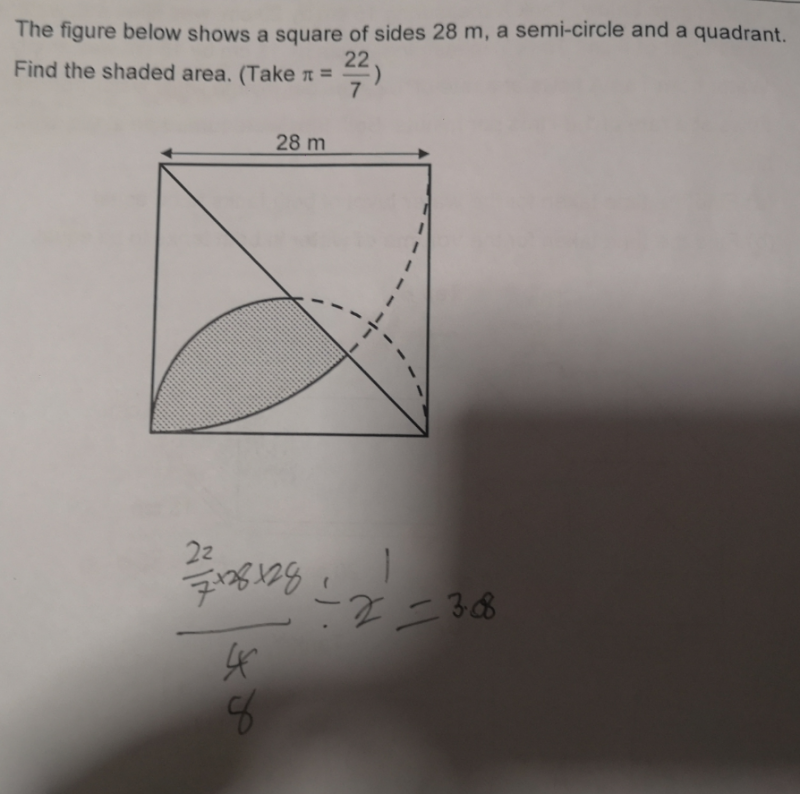# Question

By drawing another line from the bottom left corner of square to its top right, the shaded area is divided into 2 parts : upper and lower.
area of upper part: = (1/4) x (22/7) x 28/2 x 28/2) – (1/2) x (28/2) x (28/2) = 154 – 98 = 56
area of lower part = [(1/4) x (22/7) x 28 x 28 – (1/2) x 28 x 28]/2 = (616 – 392)/2 = 112
56 + 112 = 168

Ans : 168 sq m.

0 Replies 0 LikesWith reference to picture attached:

You can see the shaded area is divided into two parts by the red line.

Area of top shaded part = (Area of semi-circle DOC – Area of triangle DOC) ÷ 2
= [(1/2 x 22/7 x 14 x 14) – (1/2 x 28 x 14)] ÷ 2
= (308 – 196) ÷ 2
= 112 ÷ 2
= 56 m2

= [(1/4 x 22/7 x 28 x 28) – (1/2 x 28 x 28)] ÷ 2
= (616 – 392) ÷ 2
= 224 ÷ 2
= 112 m2

Area of whole shaded part → 56 + 112 = 168 m2

0 Replies 1 LikeSplit shaded area into 2 parts by drawing the line from top right corner to bottom left corner of square

To find the lower part:(area of quadrant – area of triangle which is half square)÷2

To find the upper part:(area of semi-circle-area of triangle which is a quarter square)÷2

0 Replies 1 Like